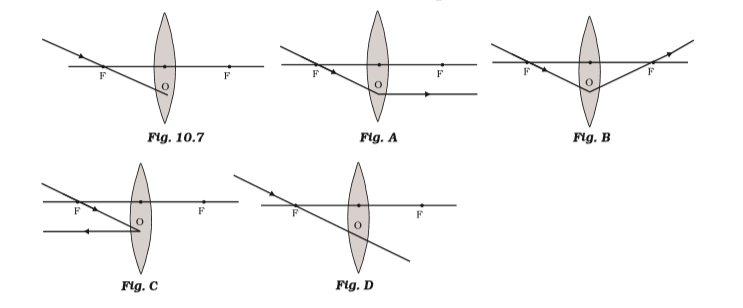# Which of the following ray diagrams is correct for the ray of light incident on a lens shown in Figure? (a) Fig. A. (b) Fig. B. (c) Fig. C. (d) Fig. D.In the convex lens, the incident ray passing through F goes parallel to the principal axis after refraction.

## Convex lens

• A convex lens is a lens with an outward curve.
• The thickness at the centre of a convex lens is more than the thickness at the edges of the lens.
• Convex lenses are converging lenses. They have the ability to converge a parallel beam of light into a point.
• The lens found in the human eye is a prime example of a convex lens.
• Another common example of a convex lens is the magnifying glass that is used to correct Hypermetropia or long-sightedness.(0)(0)